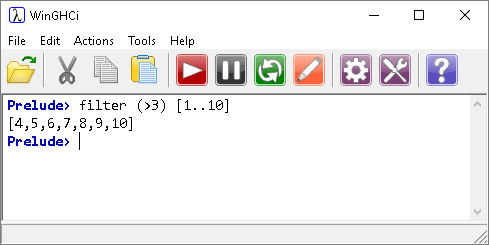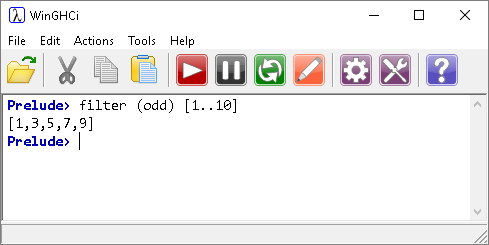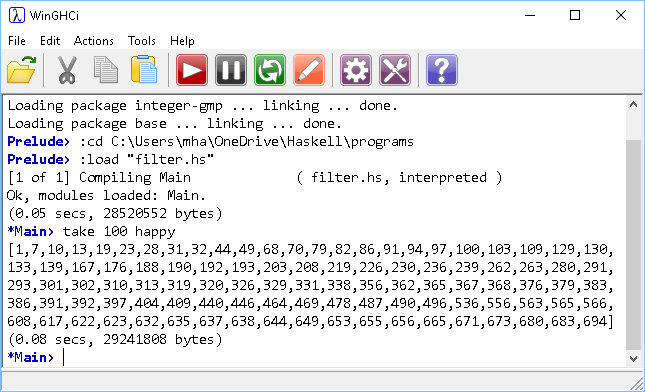## Introduction

The filter function is a higher-order function that processes a data structure, typically a list, in some order to produce a new data structure containing exactly those elements of the original data structure that match a given condition.

The difference between this and the map function is that this must take a predicate, an expression or function that evaluates to true or false.or,## Worked Example - Happy Numbers

The following example makes use of all of many of the language features described on the pages up to this one. There are a couple of extras thrown in that prove useful in Haskell programs.

To find out if a number is a happy number, we carry out the following steps.

• Take each digit in the number and square it, adding up the sum of all those squares.
• Keep repeating the process with the result until the result is either 1 (which means that the number you started with was a happy number or you keep getting a repeated cycle of numbers.

For example,

Number49
Sum of squares of digits42 + 92 = 16 + 81 = 97
Sum of squares of digits92 + 72 = 81 + 49 = 130
Sum of squares of digits12 + 32 + 02 = 1 + 9 + 0 = 10
Sum of squares of digits12 + 02 = 1 + 0 = 1
Result49 is a happy number

Another example,

Number11
Sum of squares of digits12 + 12 = 1 + 1 = 2
Sum of squares of digits22 = 4
Sum of squares of digits42 = 16
Sum of squares of digits12 + 62 = 1 + 36 = 37
Sum of squares of digits32 + 72 = 9 + 49 = 58
Sum of squares of digits52 + 82 = 25 + 64 = 89
Sum of squares of digits82 + 92 = 64 + 81 = 145
Sum of squares of digits12 + 42 + 52 = 1 + 16 + 25 = 42
Sum of squares of digits42 + 22 = 16 + 4 = 20
Sum of squares of digits22 + 02 = 4 + 0 = 4
Result11 is not a happy number

In this second example, the sequence returns to 4. If the sum of the squares of the digits is ever one of these numbers, the number is not happy. For all unhappy numbers, this sequence will be reached somehow. Checking for a result of 4 is enough to determine that a number is not happy.

Here is the program to do this, leading up to using the filter function to select from a list of positive integers, only those numbers that are happy.

```-- convert a number to a list of digits
toDigits n
| n < 1 = []
| otherwise = toDigits (div n 10) ++ [mod n 10]

-- composition of sum and toDigits functions
sumDigits = sum.toDigits

-- square the digits
squareDigits n = map (^2) (toDigits n)

-- sum of the squares of the digits
sumSquareDigits = sum.squareDigits

-- check if a number is a happy number
ishappy 1 = True                        -- sequence stops at 1
ishappy 4 = False                       -- endless cycle
ishappy n = ishappy (sumSquareDigits n)

-- happy number infinite list
happy = filter ishappy [1..]
```

Here is the program running, using the take function to select the first 100 happy numbers,Let's take apart the program a function at a time, starting with the digit separating function.

```-- convert a number to a list of digits
toDigits n
| n < 1 = []
| otherwise = toDigits (div n 10) ++ [mod n 10]

-- composition of sum and toDigits functions
sumDigits = sum.toDigits
```

The pipe characters are called guards. The last condition, otherwise catches everything not covered by one of the other guards.

Think about how we take apart the digits from our starting value, n. The rightmost digit is the remainder when n is divided by 10. To remove that digit, we do integer division by 10. We keep repeating this process until there are no digits left. The otherwise condition is a recursive call. It is the equivalent of saying, 'the digits of n is a list consisting of the digits of n divided by 10 and ending in the remainder when n is divided by 10'. The first guard is there to end the recursion when all of the digits have been extracted.

```-- square the digits
squareDigits n = map (^2) (toDigits n)

-- sum of the squares of the digits
sumSquareDigits = sum.squareDigits
```

Here we use the map function to get a list of the squares of the digits. The other function composes this with the sum function to make for easier reading later on.

```-- check if a number is a happy number
ishappy 1 = True                        -- sequence stops at 1
ishappy 4 = False                       -- endless cycle
ishappy n = ishappy (sumSquareDigits n)
```

The ishappy function is an alternative to using guards. Again, we have recursion.

```-- happy number infinite list
happy = filter ishappy [1..]
```

Finally, we can construct an infinite list by filtering the positive integers using our ishappy function.